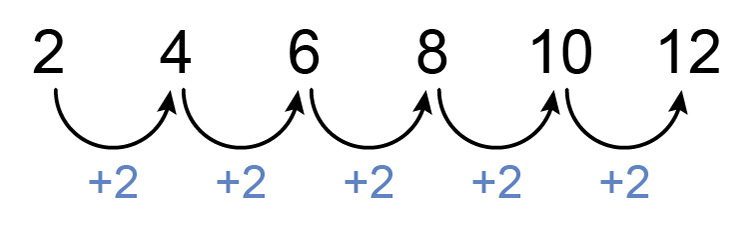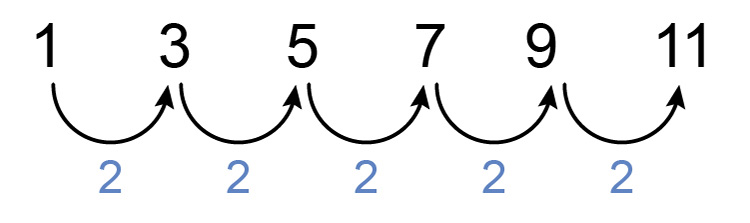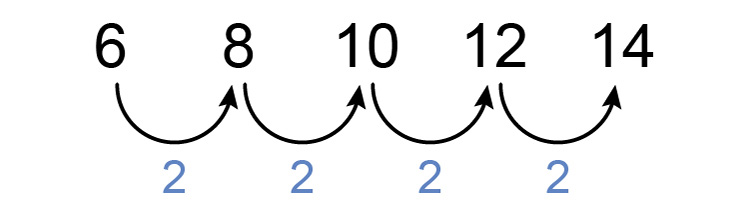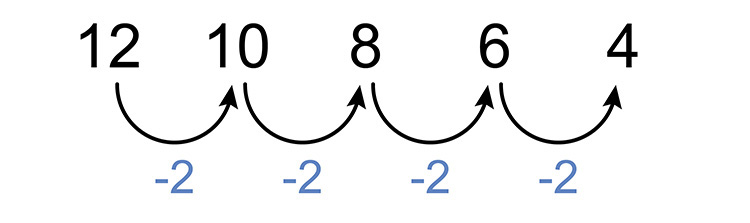# Formula for n^(th) term of a sequence - consistent difference

In order to predict the n^(th)  term of a sequenece you will need to create a formula.

For sequence patterns of consistent differences finding the n^(th)  term of a linear sequence (an addition or subtraction sequence) is worked out by.or in more detailSummary

Formula for n^(th)  term =  Difference timesn+(first term-difference)

Example 1

## Even numbers

What is the formula for the n^(th)  term for the following sequence, and what is the 11^(th)  term?

2,   4,   6,   8,   10,   12

To tackle this always find the sequence pattern.

First, see if there is a consistent difference between each number.Yes and that number is 2

Now to find the formula:

i. Find the difference and multiply by n  =2n

The difference is 2

Which we now multiply by n

Therefore this equals 2n

ii. Find the value between the first term and the consistent difference.

 First term = 2 Consistent difference = 2 Take them away = 0

So the value between the first term and the consistent difference =0

iii. Adding the two together we get the formula for this sequence as:

Formula for n^(th)  term =2n+0

Answer: The formula for the n^(th)  term =2n

NOTE:

This formula is well known as the sequence progression for even numbers.

Always check the formula is correct.

Try different values of n in the formula 2n

If n=1          therefore term =2times1=2

If n=2          therefore term =2times2=4

If n=3          therefore term =2times3=6

This is correct

Now we have the correct formula we can work out the other terms.

The 11^(th)  term would be:

If n=11          then 2n=2times11=22

Answer: The 11^(th)  term =22

Example 2

## Odd numbers

What is the formula for the n^(th)  term for the following sequence, and what is the 14^(th)  term.

1,   3,   5,   7,   9,   11

To tackle this always find the sequence pattern.

First, see if there is a consistent difference between each number.Yes and that number is 2

Now to find the formula:

i. Find the difference and multiply by n  =2n

The difference is 2

Which we now multiply by n

Therefore this equals 2n

ii. Find the value between the first term and the consistent difference.

 First term = 1 Consistent difference = 2 Take them away = -1

So the value between the first term and the consistent difference =-1

iii. Adding the two together we get the formula for this sequence as:

Formula for n^(th)  term =2n-1

Answer: The formula for the n^(th)  term =2n-1

NOTE:

This formula is well known as the sequence progression for odd numbers.

Always check the formula is correct.

Try different values of n in the formula 2n-1

If n=1          therefore term =2times1-1=1

If n=2          therefore term =2times2-1=3

If n=3          therefore term =2times3-1=5

If n=4          therefore term =2times4-1=7

This is correct

Now we have the correct formula we can work out other terms.

The 14^(th)  term would be:

If n=14          then 2n-1=2times14-1=27

Answer: The 14^(th)  term =27

Example 3

What is the formula for the n^(th)  term for the following sequence, and what is the 55^(th)  term.

6,   8,   10,   12,   14

To tackle this always find the sequence pattern.

First, see if there is a consistent difference between each number.Yes and that number is 2

Now to find the formula:

i. Find the difference and multiply by n  =2n

The difference is 2

Which we now multiply by n

Therefore this equals 2n

ii. Find the value between the first term and the consistent difference.

 First term = 6 Consistent difference = 2 Take them away = 4

So the value between the first term and the consistent difference =4

iii. Adding the two together we get the formula for this sequence as:

Formula for n^(th)  term =2n+4

Answer: The formula for the n^(th)  term =2n+4

Always check the formula is correct.

Try different values of n in the formula 2n+4

If n=1          therefore term =2times1+4=6

If n=2          therefore term =2times2+4=8

If n=3          therefore term =2times3+4=10

This is correct

Now we have the correct formula we can work out the other terms.

The 55^(th)  term would be:

If n=55          then 2n+4=2times55+4=114

Answer: The 55^(th)  term =114

Example 4

What is the formula for the n^(th)  term for the following sequence, and what is the 7^(th)  term.

12,   10,   8,   6,   4

To tackle this always find the sequence pattern.

First, see if there is a consistent difference between each number.Yes and that number is -2

Now to find the formula:

i. Find the difference and multiply by n  =-2n

The difference is -2

Which we now multiply by n

Therefore this equals -2n

ii. Find the value between the first term and the consistent difference.

 First term = 12 Consistent difference = -2 Take them away = 14

So the value between the first term and the consistent difference =14

iii. Adding the two together we get the formula for this sequence as:

Formula for n^(th)  term =-2n+14

Answer: The formula for the n^(th)  term =-2n+14

Always check the formula is correct.

Try different values of n in the formula -2n+14

If n=1          therefore term =-2times1+14=12

If n=2          therefore term =-2times2+14=10

If n=3          therefore term =-2times3+14=8

This is correct

Now we have the correct formula we can work out other terms.

The 7^(th)  term would be:

If n=7          then -2n+14=-2times7+14=0

Answer: The 7^(th)  term =0